Create a new printableGeometry
Math Worksheets

Sample - Click above to make a new math worksheet (PDF).
 Name _____________________________Date ___________________
Lines and Angles
Complete.
 1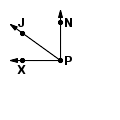Name a pair of complementary angles.
 2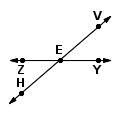Name a pair of adjacent angles.
 3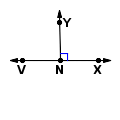Name a pair of supplementary angles.

Complete.
 4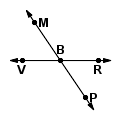Name a pair of adjacent angles.
 5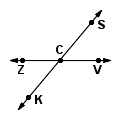Name a pair of supplementary angles.
 6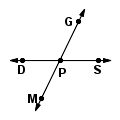Name a pair of vertical angles.

Find the measure of the angle.
 7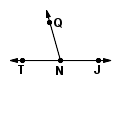m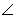TNQ = _________mJNQ = 1058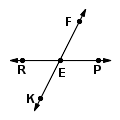mPEF = _________mREK = 639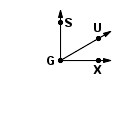mSGU = _________mUGX = 29Key #2
 aKey #2
Complete.
 10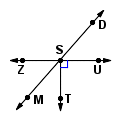Name a pair of complementary angles.
 11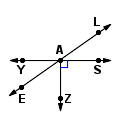Name a pair of adjacent angles.
 12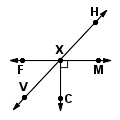Name a pair of supplementary angles.

Find the measure of the angle.
 13mMST = _________mMSZ = 4914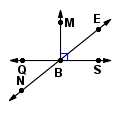mEBS = _________mNBQ = 3915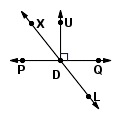mLDQ = _________mXDP = 52Fill in the blank with an angle.
16.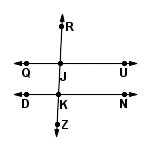QU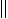DNQJR and _________ are corresponding angles
17.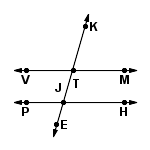VMPHKTM and _________ are corresponding angles
18.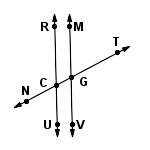RUMVUCG and _________ are vertical angles

 Key #2
 aKey #2
Find the measure of the angle.
19.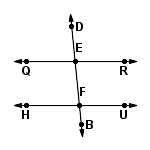QRHU
mDER = _________
mHFB = 8420.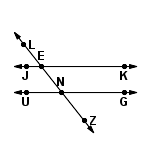JKUG
mENG = _________
mKEN = 12821.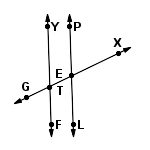YFPL
mGTY = _________
mGTF = 74Find the measure of the angle.
 22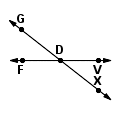mFDX = _________mFDG = 3823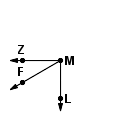mLMF = _________mFMZ = 3124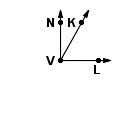mNVK = _________mKVL = 60Fill in the blank with an angle.
25.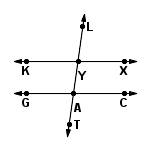KXGCLYX and _________ are alternate exterior angles
26.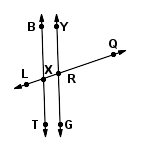BTYGXRG and _________ are vertical angles
27.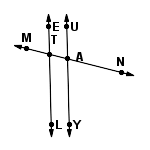ELUYETA and _________ are corresponding angles